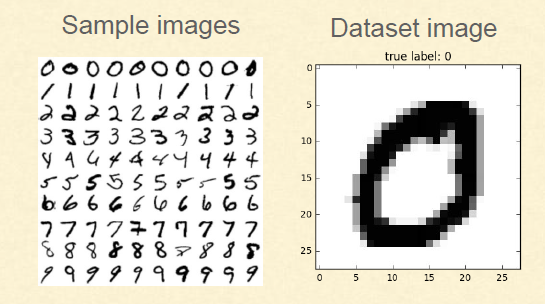# Recognizing Handwritten Digits

Original article was published by Vineet Raj Parashar on Artificial Intelligence on Medium# Dataset

● Using MNIST dataset

○ Set of 70,000 small handwritten images of digits

○ Labeled by the digits it represents

○ Can be fetched using the scikit-learn helper function

Each image: 28 X 28 pixels, 784 features

● There are 70000 such images making the dataset dimension:

○ 70000 X 784

# Fetching MNIST dataset in Scikit-Learn

>>> from sklearn.datasets import fetch_mldata

>>> mnist = fetch_mldata(“MNIST original”)

>>> X, y = mnist[“data”], mnist[“target”]

Steps

Divide dataset into training and test samples -> Train the classifier using the training dataset -> Test using test dataset -> Performance metrics (Finalize the model) -> Improve the model using error analysis.

## Training and Test dataset

● We split the data into

○ Training set — Contains 60,000 out of 70,000 samples

○ Test set — Contains 10,000 out of 70,000 samples

● We train the model on a training set

● And evaluate the performance of the model on the test set

## Dividing dataset into training and test in python:

>>> X_train, X_test, y_train, y_test = X[:60000], X[60000:], y[:60000], y[60000:]

>>> import numpy as np

>>> np.random.seed(42)

>>> shuffle_index = np.random.permutation(60000)

>>> X_train, y_train = X_train[shuffle_index], y_train[shuffle_index]

# Binary Classifier

● What is a Binary Classification?

○ Binary or binomial classification is the task of classifying the elements

of a given set into two groups (predicting which group each one

belongs to) based on a classification rule.

Classifier used: Stochastic Gradient Descent (SGD) Classifier

## Training a Binary Classifier using SGD

● Stochastic Gradient Descent (SGD) Classifier

○ Capable of handling large datasets

○ Deals with training instances independently

○ Well suited for online training

>>> from sklearn.linear_model import SGDClassifier

>>> sgd_clf = SGDClassifier(random_state=42, max_iter=10)

>>> sgd_clf.fit(X_train, y_train_5)

TEST:

>>> some_digit = X # Taking the 36,000th image

>>> sgd_clf.predict([some_digit])

array([True], dtype=bool)

## Performance measure — Methods

● Cross Validation — Accuracy

● Confusion Matrix

○ Precision

○ Recall

○ F1 score

● ROC Curve

What is cross-validation?

● It involves splitting the training set into K distinct subsets called folds,

then training and evaluating the model K times, picking a different fold for

evaluation every time and training on the other K-1 folds.

● The result is an array containing K evaluation scores.

cross_val_score:As discussed in end-to-end project session, cross_val_score() function in scikit-learn can be used to perform cross validation.

>>> from sklearn.model_selection import cross_val_score

>>> cross_val_score(sgd_clf, X_train, y_train_5, cv=3, scoring=”accuracy”)

Scoring parameter Classifier object Training data Labels No.of folds

(Here, scoring parameter is accuracy)

What is confusion matrix?

○ The general idea is to count the number of times instances of class A

are classified as class B.

○ Can be better than simple accuracy

For ‘5’ and ‘Not 5’ classifier

● The first row of this matrix considers non-5 images (the negative class):

○ 53,272 of them were correctly classified as non-5s (they are called true negatives)

○ The remaining 1,307 were wrongly classified as 5s (false positives).

Confusion matrix in Scikit Learn

>>> from sklearn.model_selection import cross_val_predict

>>> y_train_pred = cross_val_predict(sgd_clf, X_train, y_train_5, cv=3)

>>> from sklearn.metrics import confusion_matrix

>>> confusion_matrix(y_train_5, y_train_pred)

## Precision and recall

True — Positive means the classifier correctly classified the Positive class.

True — Negative means the classifier correctly classified the Negative class.

False — Positive means the classifier incorrectly classified a Negative class as Positive Class.

False — Negative means the classifier incorrectly classified a Positive class as Negative Class.

## F1 score

F1 score = harmonic mean of precision and recall

>>> from sklearn.metrics import f1_score

>>> f1_score(y_train_5, y_train_pred)